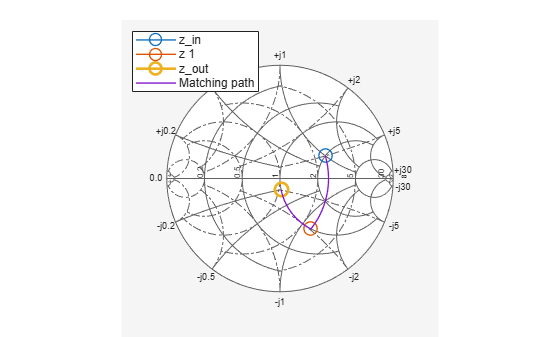# smithplot

Plot impedance transformation for selected matching network on smith chart

Since R2019a

## Syntax

``smithplot(mnobj)``
``smithplot(___,Name,Value)``

## Description

````smithplot(mnobj)` plots the impedance transformation from the source to load for the best matching network on a smith chart.```

example

````smithplot(___,Name,Value)` plots the impedance transformation from the source to load on a Smith chart with additional properties given by Name, Value pair . For instance ```smithplot (MNOBJ, 'CircuitIndex',____, 'Z0', _____)``` plots the impedance transformation for the selected matching network circuit at the characteristic impedance, `Z0` on a smith chart.Properties not specified retain their default values. NoteFor more name value pairs see, Tips. ```

## Examples

collapse all

Create a new matchingnetwork and plot the impedance transformation of the second matching network circuit in the design.

```f = 2e9; % Center frequency, Hz Zin = 150+75i; % Source impedance, Ohms Zl = 75+15i; % Load impedance, Ohms Zo = 75; % characteristic impedance of system, Ohms```

Design matching network object

```n = matchingnetwork('CenterFrequency',f,'Bandwidth',1e8, ... 'LoadImpedance',Zl,'SourceImpedance',Zin,'Components',2);```

View Circuit #2

`n.Circuit(2)`
```ans = circuit: Circuit element ElementNames: {'C' 'L'} Elements: [1x2 rf.internal.circuit.RLC] Nodes: [1 2 3] Name: 'auto_1' NumPorts: 2 Terminals: {'p1+' 'p2+' 'p1-' 'p2-'} ```

Impedance transformation of Circuit #2 on a smith chart

`hs = smithplot(n, 'Z0', Zo, 'CircuitIndex',2);`## Input Arguments

collapse all

Matching network, specified as a `matchingnetwork` object.

Data Types: `char` | `string`

### Name-Value Arguments

Specify optional pairs of arguments as `Name1=Value1,...,NameN=ValueN`, where `Name` is the argument name and `Value` is the corresponding value. Name-value arguments must appear after other arguments, but the order of the pairs does not matter.

Before R2021a, use commas to separate each name and value, and enclose `Name` in quotes.

Example: `'CircuitIndex',1`

Selected matching network, specified as a positive scalar.

Data Types: `double`

Characteristic impedance, specified as scalar in ohms.

Data Types: `double`

## Tips

• To list other property `Name,Value` pairs in `smithplot`, use `details(s)` where `s` is a `smithplot` object. You can use the properties to extract any data from the Smith chart.

• For a list of properties of `smithplot`, see .

• You can use the `smithplot` interactive menu to change the line and marker styles.

## Version History

Introduced in R2019a